# Reliability Growth Models

The exponential model can be regarded as the basic form of the software reliability growth models. For the past two decades, software reliability modeling has been one of the most active areas in software engineering. More than a hundred models have been proposed in professional journals and at software conferences, each with its own assumptions, applicability, and limitations. Unfortunately, not many models have been tested in practical environments with real data, and even fewer models are in use. From the practical software development point of view, for some models the cost of gathering data is too expensive; some models are not understandable; and some simply do not work when examined. For instance, Elbert and associates (1992) examined seven reliability models with data from a large and complex software system that contained millions of lines of source code. They found that some models gave reasonable results, and others provided unrealistic estimates. Despite a good fit between the model and the data, some models predicted the prob-ability of error detection as a negative value. The range of the estimates of the defects of the system from these models is incredibly wide ”from 5 to 6 defects up to 50,000.

Software reliability growth models can be classified into two major classes, depending on the dependent variable of the model. For the time between failures models, the variable under study is the time between failures. This is the earliest class of models proposed for software reliability assessment. It is expected that the failure times will get longer as defects are removed from the software product. A common approach of this class of model is to assume that the time between, say, the ( i - 1)st and the i th failures follows a distribution whose parameters are related to the number of latent defects remaining in the product after the (i - 1)st failure. The distribution used is supposed to reflect the improvement in reliability as defects are detected and removed from the product. The parameters of the distribution are to be estimated from the observed values of times between failures. Mean time to next failure is usually the parameter to be estimated for the model.

For the fault count models the variable criterion is the number of faults or failures (or normalized rate) in a specified time interval. The time can be CPU execution time or calendar time such as hour , week, or month. The time interval is fixed a priori and the number of defects or failures observed during the interval is treated as a random variable. As defects are detected and removed from the software, it is expected that the observed number of failures per unit time will decrease. The number of remaining defects or failures is the key parameter to be estimated from this class of models.

The following sections concisely describe several models in each of the two classes. The models were selected based on experience and may or may not be a good representation of the many models available in the literature. We first summarize three time between failures models, followed by three fault count models.

8.2.1 Jelinski-Moranda Model

The Jelinski-Moranda (J-M) model is one of the earliest models in software reliability research (Jelinski and Moranda, 1972). It is a time between failures model. It assumes N software faults at the start of testing, failures occur purely at random, and all faults contribute equally to cause a failure during testing. It also assumes the fix time is negligible and that the fix for each failure is perfect. Therefore, the software product's failure rate improves by the same amount at each fix. The hazard function (the instantaneous failure rate function) at time t i , the time between the ( i - 1)st and i th failures, is given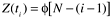where N is the number of software defects at the beginning of testing and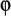is a pro-portionality constant. Note that the hazard function is constant between failures but decreases in steps offollowing the removal of each fault. Therefore, as each fault is removed, the time between failures is expected to be longer.

8.2.2 Littlewood Models

The Littlewood (LW) model is similar to the J-M model, except it assumes that different faults have different sizes, thereby contributing unequally to failures (Littlewood, 1981). Larger- sized faults tend to be detected and fixed earlier. As the number of errors is driven down with the progress in test, so is the average error size , causing a law of diminishing return in debugging. The introduction of the error size concept makes the model assumption more realistic. In real-life software operation, the assumption of equal failure rate by all faults can hardly be met, if at all. Latent defects that reside in code paths that rarely get executed by customers' operational profiles may not be manifested for years .

Littlewood also developed several other models such as the Littlewood non-homogeneous Poisson process (LNHPP) model (Miller, 1986). The LNHPP model is similar to the LW model except that it assumes a continuous change in instantaneous failure rate rather than discrete drops when fixes take place.

8.2.3 Goel-Okumoto Imperfect Debugging Model

The J-M model assumes that the fix time is negligible and that the fix for each failure is perfect. In other words, it assumes perfect debugging. In practice, this is not always the case. In the process of fixing a defect, new defects may be injected. Indeed, defect fix activities are known to be error-prone . During the testing stages, the percentage of defective fixes in large commercial software development organizations may range from 1% or 2% to more than 10%. Goel and Okumoto (1978) proposed an imperfect debugging model to overcome the limitation of the assumption. In this model the hazard function during the interval between the ( i - 1)st and the i th failures is givenwhere N is the number of faults at the start of testing, p is the probability of imperfect debugging, and l is the failure rate per fault.

8.2.4 Goel-Okumoto Nonhomogeneous Poisson Process Model

The NHPP model (Goel and Okumoto, 1979) is concerned with modeling the number of failures observed in given testing intervals. Goel and Okumoto propose that the cumulative number of failures observed at time t, N ( t ), can be modeled as a nonhomogeneous Poisson process (NHPP) ”as a Poisson process with a time-dependent failure rate. They propose that the time-dependent failure rate follows an exponential distribution. The model is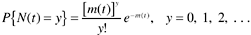where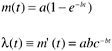In the model, m ( t ) is the expected number of failures observed by time t; l ( t ) is the failure density; a is the expected number of failures to be observed eventually; and b is the fault detection rate per fault. As seen, m ( t ) and l ( t ) are the cumulative distribution function [ F ( t) ] and the probability density function [ f ( t) ], respectively, of the exponential function discussed in the preceding section. The parameters a and b correspond to K and l . Therefore, the NHPP model is a straight application of the exponential model. The reason it is called NHPP is perhaps because of the emphasis on the probability distribution of the estimate of the cumulative number of failures at a specific time t, as represented by the first equation. Fitting the model curve from actual data and for projecting the number of faults remaining in the system, is done mainly by means of the mean value, or cumulative distribution function (CDF).

Note that in this model the number of faults to be detected, a, is treated as a random variable whose observed value depends on the test and other environmental factors. This is fundamentally different from models that treat the number of faults to be a fixed unknown constant.

The exponential distribution assumes a pattern of decreasing defect rates or failures. Cases have been observed in which the failure rate first increases and then decreases. Goel (1982) proposed a generalization of the Goel-Okumoto NHPP model by allowing one more parameter in the mean value function and the failure density function. Such a model is called the Goel generalized nonhomogeneous Poisson process model;where a is the expected number of faults to be eventually detected, and b and c are constants that reflect the quality of testing. This mean value function and failure density function is actually the Weibull distribution, which we discussed in Chapter 7. When the shape parameter m (in the Goel model, it is c ) equals 1, the Weibull distribution becomes the exponential distribution; when m is 2, it then becomes the Rayleigh model.

8.2.5 Musa-Okumoto Logarithmic Poisson Execution Time Model

Similar to the NHPP model, in the Musa-Okumoto (M-O) model the observed number of failures by a certain time, t , is also assumed to be a nonhomogeneous Poisson process (Musa and Okumoto, 1983). However, its mean value function is different. It attempts to consider that later fixes have a smaller effect on the software's reliability than earlier ones. The logarithmic Poisson process is claimed to be superior for highly nonuniform operational user profiles, where some functions are executed much more frequently than others. Also the process modeled is the number of failures in specified execution-time intervals (instead of calendar time). A systematic approach to convert the results to calendar-time data (Musa et al., 1987) is also provided. The model, therefore, consists of two components ”the execution-time component and the calendar-time component.

The mean value function of this model is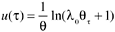where l is the initial failure intensity, and q is the rate of reduction in the normalized failure intensity per failure.

8.2.6 The Delayed S and Inflection S Models

With regard to the software defect removal process, Yamada et al. (1983) argue that a testing process consists of not only a defect detection process, but also a defect isolation process. Because of the time needed for failure analysis, significant delay can occur between the time of the first failure observation and the time of reporting. They offer the delayed S-shaped reliability growth model for such a process, in which the observed growth curve of the cumulative number of detected defects is S-shaped. The model is based on the nonhomogeneous Poisson process but with a different mean value function to reflect the delay in failure reporting,where t is time, l is the error detection rate, and K is the total number of defects or total cumulative defect rate.

In 1984, Ohba proposed another S-shaped reliability growth model ”the inflection S model (Ohba, 1984). The model describes a software failure detection phenomenon with a mutual dependence of detected defects. Specifically, the more failures we detect, the more undetected failures become detectable. This assumption brings a certain realism into software reliability modeling and is a significant improvement over the assumption used by earlier models ”the independence of faults in a program. Also based on the nonhomogeneous Poisson process, the model's mean value function is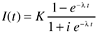where t is time, l is the error detection rate, i is the inflection factor, and K is the total number of defects or total cumulative defect rate.

The delayed S and inflection S models can be regarded as accounting for the learning period during which testers become familiar with the software at the beginning of a testing period. The learning period is associated with the delayed or inflection patterns as described by the mean value functions. The mean value function (CDF) and the failure density function (PDF) curves of the two models, in comparison with the exponential model, are shown in Figure 8.3. The exponential model assumes that the peak of defect arrival is at the beginning of the system test phase, and continues to decline thereafter; the delayed S model assumes a slightly delayed peak; and the inflection S model assumes a later and sharper peak.

Figure 8.3. Exponential, Delayed S, and Inflection S Models ”PDF (left ) and CDF (right )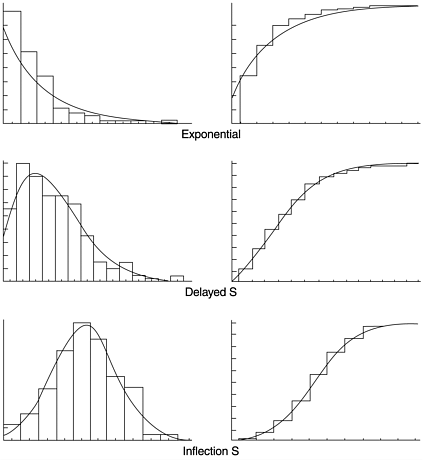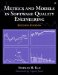Metrics and Models in Software Quality Engineering (2nd Edition)
ISBN: 0201729156
EAN: 2147483647
Year: 2001
Pages: 176

Similar book on Amazon